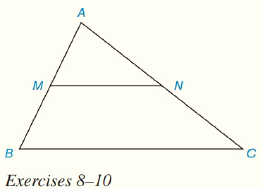Chapter 4.CT, Problem 9CTElementary Geometry For College St...

7th Edition
Alexander + 2 others
ISBN: 9781337614085

Solutions

Chapter
SectionElementary Geometry For College St...

7th Edition
Alexander + 2 others
ISBN: 9781337614085
Textbook Problem

In Δ A B C , M is the midpoint of A B ¯ and N is the midpoint of A C ¯ . If MN=7.6 cm, find BC.To determine

To Find:

Length of BC.

Explanation

Given:

M is the midpoint of AB¯ and N is the midpoint of AC¯

Calculation:

The line joining the midpoints of two sides of a triangle is parallel to the third side

Still sussing out bartleby?

Check out a sample textbook solution.

See a sample solution

The Solution to Your Study Problems

Bartleby provides explanations to thousands of textbook problems written by our experts, many with advanced degrees!

Get Started

Find the general indefinite integral. (1+rr)2dr

Single Variable Calculus: Early Transcendentals, Volume I

Expand each expression in Exercises 122. x(3x+y)

Finite Mathematics and Applied Calculus (MindTap Course List)

If f(x) = 5x, show that f(x+h)f(x)h=5x(5h1h)

Single Variable Calculus: Early Transcendentals

Which curve is simple but not closed?

Study Guide for Stewart's Multivariable Calculus, 8th

True or False: The function graphed at the right is one-to-one.

Study Guide for Stewart's Single Variable Calculus: Early Transcendentals, 8th